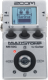# bridge of sighs

Discussion in 'Zoom MS-50G' started by wax, Mar 26, 2019.

1. bridge of sighsDevice: Zoom Ms-50g
Firmware: 3.00

Name on device: RK~1~ `9
Optimized for: Phones/Speaker

Effects chain:neck

Effect: "Comp" (Dynamics / Filter), active - "yes"
"Sense" = 8
"Tone" = 6
"Level" = 100
"ATTCK" = Slow

Effect: "OverDrive" (Overdrive / Distortion), active - "yes"
"Gain" = 94
"Tone" = 41
"Level" = 98

Effect: "Delay" (Delay / Reverb), active - "no"
"Time" = 499
"F.B" = 0
"Mix" = 32
"HiDMP" = 10
"P-P" = MONO
"Level" = 100
"Tail" = On

Effect: "MS 1959" (Amp simulator), active - "yes"
"Gain" = 29
"Tube" = 30
"Level" = 150
"Trebl" = 68
"Middl" = 33
"Bass" = 81
"Prese" = 51
"CAB" = MS 1959 4x12
"OUT" = LINE

Effect: "TheVibe" (Modulation / Sfx), active - "yes"
"Speed" = 16
"Depth" = 48
"Bias" = 48
"Wave" = 54
"Mode" = VIBRT
"Level" = 100

Effect: "GraphicEQ" (Dynamics / Filter), active - "yes"
"160Hz" = 0
"400Hz" = 0
"800Hz" = -1
"3.2kHz" = 5
"6.4kHz" = 1
"12kHz" = 0
"Level" = 100

Note: This is a patch file, you will need to download and install the ToneLib-Zoom software to use the patch.

File size:
433 bytes
Views:
235